Latest Banking jobs   »

# Reasoning Quiz For Bank of Baroda SO 2023 -19th May

Directions (1-3): Study the information carefully and answer the questions given below.
A person starts walking in north direction from point P, after walking 15m he reached at point A, then he takes right turn and walks 7m to reach at point Q. After that he walks in south direction and walks 10m to reach at point M. Point B is 5m east of point M. Point R is 22m north of point B. Point C is 10m west of point R.

Q1. What is the direction of point R with respect to person’s initial position?
(a) North
(b) West
(c) South-West
(d) North-East
(e) None of these

Q2. What is the shortest distance between point Q and point R?
(a) 13m
(b) 25m
(c) 20m
(d) 17m
(e) 22m

Q3. What is the direction of point P with respect to point B?
(a) South-East
(b) North-West
(c) South-West
(d) East
(e) None of these

Directions (4-5): Study the following information carefully and answer the questions which follow:
A person starts walking from point P in south direction, walks 10m and reached at point Q. From point Q, he takes two consecutive right turns and walks 12m and 8m respectively. Now he takes left turn and walks 5m to reach at point R, then finally takes a left turn and walks 16m to reach at point S.

Q4. What is the direction of point P with respect to final point?
(a) North-east
(b) South-west
(c) North-west
(d) East
(e) None of these

Q5. If O is the midpoint of R and S, then what is the direction of Q with respect to O?
(a) West
(b) North
(c) North-east
(d) East
(e) None of these

Directions (6-10): In each of the questions below, some statements are given followed by two conclusions numbered I and II. You have to take the given statements to be true even if they seem to be at variance with commonly known facts. Read all the conclusions and then decide which of the given conclusions logically follows from the given statements disregarding commonly known facts.

Q6. Statements: All Windows are Pastes.
All Pinks are Injects.
Only a few Pink are Windows.
Conclusions: I. All Paste being Inject is a possibility.
II. Some Pastes are Pink.
(a) If only conclusion I follows.
(b) If only conclusion II follows.
(c) If either conclusion I or II follows.
(d) If neither conclusion I nor II follows.
(e) If both conclusions I and II follow.

Q7. Statements: Some Pockets are Socks.
Only a few Socks are New.
No New is a Pocket.
Conclusions: I. All New being Socks is a possibility.
II. Some Socks are not Pockets.
(a) If only conclusion I follows.
(b) If only conclusion II follows.
(c) If either conclusion I or II follows.
(d) If neither conclusion I nor II follows.
(e) If both conclusions I and II follow.

Q8. Statements: Some money is rent.
Some bonus is principal.
No sum is bonus.
No rent is bonus.
Conclusions: I. No money is Principle.
II. All rent being sum is not possible.
(a) If only conclusion I follows.
(b) If only conclusion II follows.
(c) If either conclusion I or II follows.
(d) If neither conclusion I nor II follows.
(e) If both conclusions I and II follow.

Q9. Statements: Only a few Silvers are Golden.
All Golden are Platinum.
All Platinum are Iron.
Conclusions: I. Some Platinums are Silver.
II. Some Iron are not Silver.
(a) If only conclusion I follows.
(b) If only conclusion II follows.
(c) If either conclusion I or II follows.
(d) If neither conclusion I nor II follows.
(e) If both conclusions I and II follow.

Q10. Statements: Only a few Friends are Relatives.
All Relatives are Family.
No Family is Parent.
Conclusions: I. Some Friends are not Relatives.
II. No Relatives are Parents.
(a) If only conclusion I follows.
(b) If only conclusion II follows.
(c) If either conclusion I or II follows.
(d) If neither conclusion I nor II follows.
(e) If both conclusions I and II follow.

Solutions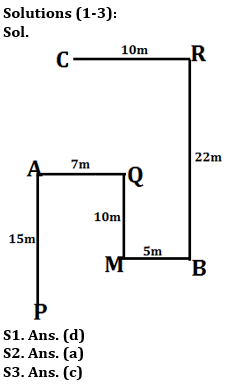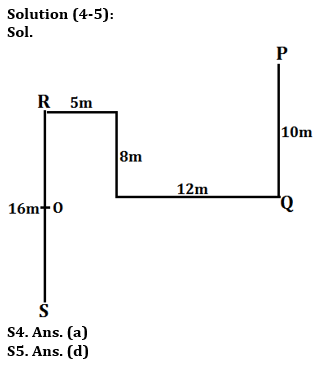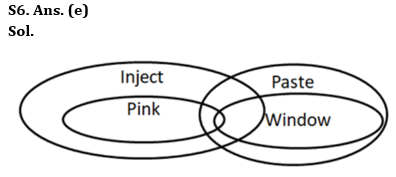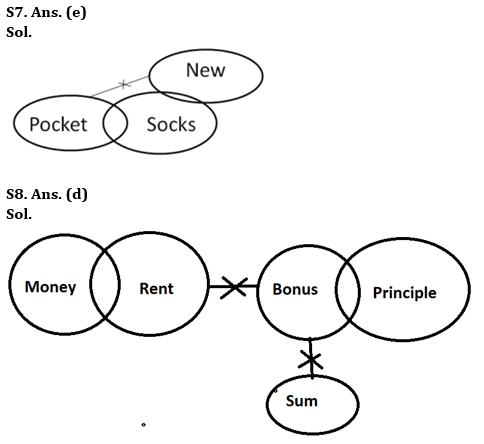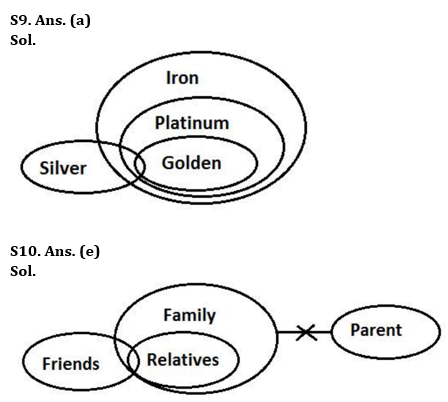## FAQs

### What is the selection process of Bank of Baroda AO 2023?

The selection process of Bank of Baroda AO consists of Online exam and Interview

#### Congratulations!Union Budget 2023-24: Free PDF